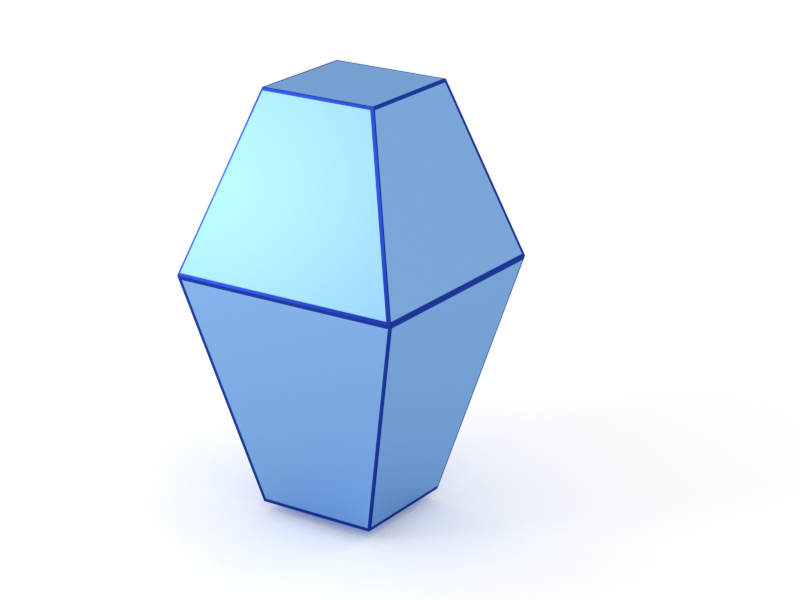### Bipyramid4

A compound of two truncated pyramids with a common square base and opposite orientations.#### Constructor

Bipyramid4(L, H, r_H, alpha)


Parameters:

• L, edge length of the shared quadratic base
• H, height of the bottom pyramid
• r_H, height ratio $r_{H}$ between the top and bottom pyramid
• alpha, dihedral angle $\alpha$ between the base and a side face.

Constraint:

$H \le \dfrac{\tan\alpha}{2} L \quad$ and $\quad r_{H} H \le \dfrac{\tan\alpha}{2} L$

#### Usage

As for any other Form factor.

#### Implementation

Class Bipyramid4 inherits from the interface class IFormFactor .

Form factor computation is based on the generic form factor of a polyhedron provided by libformfactor .

Volume has been validated against $$V=\dfrac{L^3\tan\alpha}{6}\Big[2-\Big(1-\dfrac{2H}{L\tan\alpha}\Big)^3-\Big(1-\dfrac{2r_{H}H}{L\tan\alpha}\Big)^3\Big].$$

More special:

#### Example

Scattering by uncorrelated, oriented bipyramids for horizontal incidence. Rotation around $z$ axis:Generated by Examples/ff/Bipyramid4.py .

#### History

Was incorrectly named “Cuboctahedron” up to BornAgain 1.19.

Agrees with “Cubooctaedron” form factor of IsGISAXS [manual, Eq. 2.33; Renaud 2009, Eq. 218], except for different parametrization $L = 2R_{IsGISXAXS}$. Since BornAgain-1.6 implemented using the generic polyhedron form factor [Wuttke 2021].